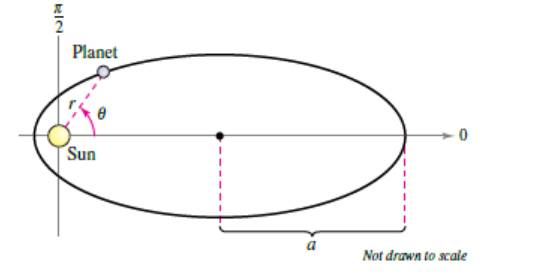Chapter 10.6, Problem 62E

Chapter
Section
Textbook Problem

# Planetary Motion The planets travel in elliptical orbits with the sun as a focus, as shown in the figure.(a) Show that the polar equation of the orbit is given by e = ( 1 − e 2 ) a 1 − e cos θ where e is the eccentricity.(b) Show that the minimum distance (perihelion) from the sun to the planet is r = a ( 1 − e ) and the maximum distance (aphelion) is r = a ( 1 + e ) .

(a)

To determine

To Prove:

That the polar equation of the orbit of the planet is r=(1e2).a1ecosθ, where e is its eccentricity.

Explanation

Given: The planets travel in an elliptical orbit with the sun as its focus, as shown in the figure below:

Formula Used:

The polar equation of curve with eccentricity e is r=ed1+ecosθ

Proof:

Consider the provided figure as:

g

According to the Kepler’s Law:

A planet revolves around the sun in an elliptical orbit with the sun at one of its focus.

The line joining the sun to a planet sweeps out equal areas in equal times.

The square of the period of revolution of planet is proportional to the cube of length of major axis of its orbit.

It is known that equation of an ellipse in terms of eccentricity e and its semi-major axis a is, a2=e2d2(1e2)2

The distance d from the focus to the directrix in terms of a is:

d

(b)

To determine

To Prove:

That the minimum distance (perihelion) from the sun to a planet is r=a(1e) and maximum distance aphelion is r=a(1+e).

### Still sussing out bartleby?

Check out a sample textbook solution.

See a sample solution

#### The Solution to Your Study Problems

Bartleby provides explanations to thousands of textbook problems written by our experts, many with advanced degrees!

Get Started

#### Find more solutions based on key concepts